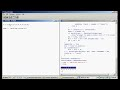### Finding the Mode of a Variable in RQuick Tip:

Given some vector or matrix "x"
x <- c(1,2,2,2,3,4,5,6,7,8,9)

The following code returns the mode(s)
temp <- table(as.vector(x))

### The second row in "temp" counts how many occurrences of each value. So there's be "1"   under the values 1,3,4,5,6,7,8 & 9. And a "3" under the value 2.

names(temp)[temp == max(temp)]

### #this returns the names of the values that have the highest count in temp's second row.

Since the mode is the value(s) that occur most frequently in a vector or matrix, this line returns the mode.

 "2"

Full Details and Instructions:

Comments